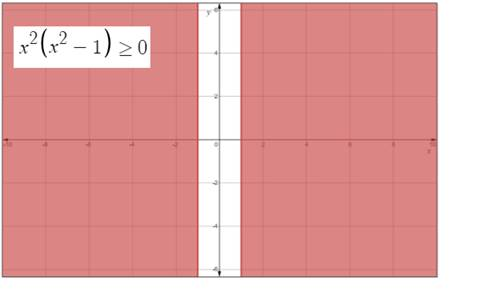# the nonlinear inequality. Express the solution using interval notation and graph the solution set.### Precalculus: Mathematics for Calcu...

6th Edition
Stewart + 5 others
Publisher: Cengage Learning
ISBN: 9780840068071### Precalculus: Mathematics for Calcu...

6th Edition
Stewart + 5 others
Publisher: Cengage Learning
ISBN: 9780840068071

#### Solutions

Chapter 1.7, Problem 54E
To determine

## To solve: the nonlinear inequality. Express the solution using interval notation and graph the solution set.

Expert Solution

[,1)(1,] .

### Explanation of Solution

Given:

The given inequality is x2(x21)0 .

Concept used:

Guidelines for solving nonlinear inequality:

1. Move all terms to one side.
2. Factor the non-zero side of the inequality.
3. Find the value for which each factor is zero. The number will divide the real lines into interval. List the interval determined by these numbers
4. Make a table or diagram by using test values of the signs of each factor on each interval. In the last row of the table determining the sign of the product of these factors.
5. Determine the solution of the inequality from the last row of the sign table.

Calculation:

As the given inequality is x2(x21)0 .

Since all the terms in left hand side, then

x2(x21)0{giveninequality}x2(x1)(x+1)0{factor}

The factor on the left-hand side are x2,(x1), and (x+1) . These factors are zero when x=0,1,1 respectively. The number divide the real lines into four intervals.

[,1)[1,0],[0,1],(1,]

Now, make a table by using test values of the signs of each factor on each interval.

 (−∞,−1) (−1,0) (0,1) (1,∞) x2 + + + + (x+1) − + + + (x−1) − − − + x2,(x−1), and (x+1) + − − +

Hence,the solution set is [,1)(1,] .

The graph of the non-linear inequality x2(x21)0 is:### Have a homework question?

Subscribe to bartleby learn! Ask subject matter experts 30 homework questions each month. Plus, you’ll have access to millions of step-by-step textbook answers!